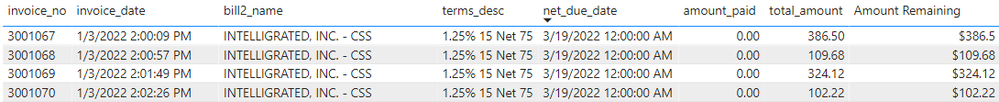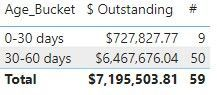cancel
Showing results for
Did you mean:Frequent Visitor

## Creating an Aging Bucket

Hello all,

I am new to PowerBI and am looking to create an AR Aging bucket. I have pulled all the relevant information from our SQL server, but cannot figure out how to create calculate columns based on the net_due_date field.

We are looking to create 30, 60, 90, and over 90 day aging buckets for reporting that would pull the amount_remaining field to the appropriate buckets based on age.

What would the formulas look like to create these buckets?

Below are the current fields that I have for the table.1 ACCEPTED SOLUTIONSuper User

@Celliott04 what is your definition for "age"?  If it is net_due_date - invoice_date, then a calculated columns something like below might work:

``````Age_Bucket =
VAR _Age = DATEDIFF('Table'[invoice_date],'Table'[net_due_Date], DAY)
VAR _Result =
SWITCH(
TRUE(),
_Age < 30, "0-30 days",
_Age >= 30 && _Age < 60, "30-60 days",
_Age >= 60 && _Age < 90, "60-90 days",
_Age >= 90, "90+ days"
)
Return
_Result``````
3 REPLIES 3Frequent Visitor

ebeery, Thanks so much for the Age_Bucket code. It works great!Super User

Hi,

We can use the CALCULATE() and FILTER() functions in a calculated column formula to get your desired result.  I can offer more help if you share the link from where i can download your PBI file.

Regards,
Ashish Mathur
http://www.ashishmathur.comSuper User

@Celliott04 what is your definition for "age"?  If it is net_due_date - invoice_date, then a calculated columns something like below might work:

``````Age_Bucket =
VAR _Age = DATEDIFF('Table'[invoice_date],'Table'[net_due_Date], DAY)
VAR _Result =
SWITCH(
TRUE(),
_Age < 30, "0-30 days",
_Age >= 30 && _Age < 60, "30-60 days",
_Age >= 60 && _Age < 90, "60-90 days",
_Age >= 90, "90+ days"
)
Return
_Result``````